### Scribe Post for April 14-15, 2010

Thursday, April 15, 2010

Yesterday April 14th we learned about linear equations and to create tables of values. Mr. Backe also told us about www.mathplayground.com and play some Math games.

He introduced Chapter 6 with some new words:
linear relation-A relation that appears as a straight line when graphed.
linear equation-An equation whose graph is a straight line.
interpolate-To estimate the value between two given values.
extrapolate-To find values that is not given

Tables of Values and linear equations
First thing to remember is that x is always the independent and y is the dependent.Here's an example of a table of values in our classTo get the linear equation you have find the difference in each number in the y column. In this table it is 3, because it adds 3 in each row in the y column. And why -2, here's an example: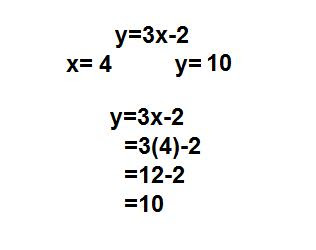Practice Question from class1. How many circles are in figure 7?2.83 circles is what figure number?Today April 15th we just corrected our tests and started to do work which is:
6.1
CYU # 2
Practise-# 4,6,7,9,10
Apply-All
Extend-# 15
6.1 Workbook
6.1 Extra Practice

### Scribe Post for April 8th, 2010

Thursday, April 8, 2010

Division of Polynomials

Today in class Mr. Backe gave us these examples.

8x(squared)/4x=2x 8x(squared)+4x/4x=2x+1

14x(cubed)+7x(squared)=2x(squared)+x

36x(squared)-12x/-4x=-9x+3 28x(squared)+21x+14/7
=4x(squared)+3x+2

Homework for tonight is:
Textbook 7.3

• All S.Y.K
• C.Y.U #2
• Practice #8 and 9
• All of Apply
• All of Extend
• 7.3 Extra Practice and 7.3 Workbook
• Chapter 7 Reveiw
• Chapter 7 test (textbook) or Hand-out test
• Chapter 7 Self Check
• Polynomial Puzzle (To hand in with Stash-It)

There is a test on Tuesday April 13, 2010

The Next Scribe is.......................................................................................Nicole N

### Scribe Post for April 5, 2010

Monday, April 5, 2010

Hi guys, it's Renz P. So here's the things we did today.

We just started 7.2 [WorkBook] it's :Here are some examples Mr. Backe' gave us.

1.]OR2.]3.]and we got a HOMEWORK too, by the way.

• READ page 264-268
• DO ALL Show-You-Know
• Check Your Understanding *Number 2,3*
• Practice 4 or 5, 6 or 7, 8 or 9 and 12 , 13
• Apply : ALL
• Extend : 19 , 20

So that's all for today (: .
Sorry for the mistakes and @Mr.Backe' sorry for posting late.
:3

### March 25, 2010 Scribe Post

Thursday, March 25, 2010

Today we didn't really do a lot, we finished our homework and go0t started on our new homework which was:
-Read page 254 to 259
-7.1 check you're understanding number 2
-Practice number 3,5,7,9,10,12,15 and 16
-Apply number 17 to 23
-Extend one of 24 to 27
We also have to turn in our stash-its with:
-Chapter 5 Self Evaluation
-Similarity Test Signed
-Chapter 5 Test Signed
-One Of The Two Chapter 5 Challenges
And that's all we did in class so get ready for the dance!

Monday, March 22, 2010

Math class today was pretty simple. We just went over our test, got homework and went over some definition words for Chapter 7.

These are Today's notes:(with pictures)

Monomial ----> give three examples using variables for at least two.
3x, -4t, 7s,

Binomial ----> give three examples with a constant.
4x+19, 4x + 13x2, 3x - 2y

Polynomial ----> an algebraic expression with four or more terms.
Ex: -4x2 + 4x + 3y + 4

Distributive property:
a (x+y) = ax + ay
a (x-y) = ax - ay
-a (x+y) = -ax - ay
-a (x-y) = -ax - ay

(Sorry the picture button doesn't work i'll edit when it works)

The next scribe is Jonathan.

### March 16, 2010. Scribe Post!

Tuesday, March 16, 2010

Hey guys, so today in math we had a test review so in this scribe im going to talk about what will be on the test.

First off lets name the parts in this number.So 7 is the Numerical Co-Efficient
The X is the Varible
The 2 is the exponent
and the constant is -9, when your talking about constants you have to put the whole thing.

Alright now its time to learn aboot LIKE TERMS!!

Like terms are 2 or more numbers that go together. The reason they go together is because they have the same variable and the same degree. Lets try an example shall we?

Which Numbers are Like Terms?

7x + 5y + 9xy + 4y

The like terms are 5y and 4y because they have the same variable which is "Y" and the same degree which is 1.

Now lets do a question like one we'll probably get on the test.

(7x + 4y + 8) - (3 + 6x -8y)

The first step we have to do is remove the brackets, now you think it would be

7x + 4y +8 -3 +6x -8y but you would be wrong if you thought that the correct answer is:

7x + 4y + 8 -3 -6x +8y. That is the correct way to write it, the reason you write it like that is because the symbol seperating the brackets is a "-" symbol so you have to change each number to its Additive Inverse.

An additive inverse is the opposite of a number. For example the additive inverse of
-1 would be 1 heres some more examples:
-6xy +6xy
7x-7x
-9y + 9y

Those are all additive inverses

Thats really all I can do for a scribe right now because the "import picture button" decided to stop working so I'll update this scribe once the "import picture button" decides to work. Sorry for the inconvienence.

### March 13, 2010 Scribe Post (:

Saturday, March 13, 2010

Hewoo people of earth today I'm going to talk about what we did in math today.

Well the student teacher person went over LIKE TERMS. So these are some information on like terms.
• They must have the same VARIABLE and DEGREE.
• They can be grouped.
• They can be +,-,x,÷

We also used algebra tiles to figure some of these questions out.

For this question we would have to find all the like terms. In other words put all the "ANIMALS" together.

Next Step:

This is when all of the " ANIMALS " .

Next Step:

For this step we had to put the animals together again and rearrange it. We have to use like terms to rearrange. ( look for the variables and the one that has the greatest degree).
and i think that was it for that math class. Sorry if i missed anything. Please tell me if i did.
HOMEWORK:
Read 5.3
Check your Understanding # 1,3
Practice # 5,8,10-17,19,20,22,27,29
Why is it good to play cards in a graveyard?(blue sheet)
Daffynition Decoder (blue sheet)
The green Booklet
This is all due for Tuesday.

### March 3, 2010 Scribe

Wednesday, March 3, 2010

Hey guys, reNz P. here! bringing you , the things that we did today in math class.
(: Guess what? We just started a new unit. and its UNIT 5.

and it is all about:So guys, now lets try to ANSWER this.

25n = _____
495x + 18d + 16o = ________
6e+w+21 = ________
rp+is+cu+73 = _______
906 + s + d + b + 3st = _______

POST a
COMMENT for YOUR ANSWER.(: that's it for today guys, hoping that the next scribe will be way more better than this.

I am SO SO SORRY for all the MISTAKES ):.

AND DON'T FORGET OUR HOMEWORK.

It's the chapter 5 in our workbook. (:hehehe *peace*

byee guys. Don't forget to comment.

### Blog Post for March 2, 2010

Tuesday, March 2, 2010

Hey guys what we did is we answered numbers 11, 14 and 15 on our Textbook chapter 4.4. If you don't have your Textbook, click HERE(http://ebook.mytextbook.ca/index.php).
Username is spmathgr9+(your textbook number)@gmail.com. I'm sure you know the password.

Here are the answers:
11. Each side is 12cm long.
14. The scale factor is 7.5
15. The new tank will hold 770 liters of water.

Our assignment is to answer Chapter 4 review and study for our test.
I will give you the answers for #1 to #4 in chapter review.

1. A POLYGON is a closed figure with sides that are line segments.
2. SIMILAR figures have equal corresponding angles and proportional corresponding side lengths.
3. The SCALE FACTOR is the constant amount my which any dimension of a shape is enlarged or reduced.
4. A PROPORTION is a statement that says two ratios are equal.

Monday, March 1, 2010

Monday, March 1 2010
Hello 9-06 today in math class we did 4.4 practice
#3 to 15 that's do tomorrow.
If you don't have Textbook you can go to website
www.mytextbook.ca there you can do your Homework.

Sorry my Scribe is short because we didn't do much this
morning.

Math Test on Wendesday.
L.A or Social Studies tomorrow.

Next scribe is Jericho Toledo.

### Blog Post for February 26, 2010

Sunday, February 28, 2010

Today in Math we watched a video about Scale factor, Similarity, and Proportions. Here is the link for the video: Scale factor, Similarity, and Proportions are related concepts2

# Now I will try to explain what I learned from the video, because its kinda hard to understand. As said in the video: Similar Figures have corresponding angles that are congruent and their corresponding sides are proportional. Corresponding means that the angles are matching and congruent means equal.This polygons are equal, but how do you know if it is equal. First you need to get the scale factor of the polygons.The first one is dividing the scale factors from the sides of the two polygons. If the answer is the same it means that the sides are equal.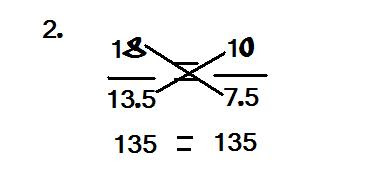The second way is multiplying the scale factors. You can multiply the scale factors any way you want, the answer would always be the same.

My post today is short, but I will try to update whenever I can. The next scribe is MARK ABAYA.

### Blog post for February,22,2010

Monday, February 22, 2010

Today in mathematics we got our stash its and journals back which was quite the horror and also our term marks which wasnt very pleasant either. But after the horrid stuff we got down to business with triangles and what not. This is what we've talked about this class:

Triangles vocabulary:
1.Scalene triangle - A triangle with no two sides of equal length.This is a scalen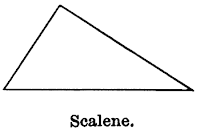e triangle

3.Isosceles triangle - A triangle with two equal sides.This is a Isosceles triangle

4.Equilateral triangle - A triangle has three sides, each one the same length. The three angles are also of equal measure.This is an equilateral triangle

5.Acute triangle - A triangle whose interior angles are all acute (less than 90 degrees)This is an Acute triangle

6.Obtuse triangle - A triangle that contains an obtuse interior angle.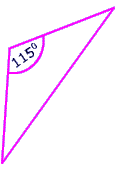This is an Obtuse triangle.

7.Equiangular triangle - A triangle in which each interior angle is identical, and each one will measure 60 degrees.This is an Equiangular triangle.

8.Right-angle triangle - A right-angle triangle or a R.A.T. is a triangle with one right angle.This is a Right-angle Triangle or a R.A.T.

Those are all the Vocabulary words from friday that we've talked about today. then after all that we then went to work on reviewing our triangle work last friday such as the formulas and how to figure out a side of a triangle and many more.

These are the examples from today: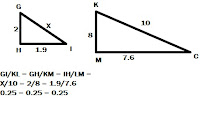So those are the three examples for today that we talked about and worked on. So now this is the homework:
1.Extra practice sheet 4.3
2.Workbook 4.3
3.Stuff from friday:
*Workbook 4.2
*Workbook get ready
(all due tomorrow)

OH and also the next scribe is ...... ...... ...... ..... ...... ..... ..... Jethro Toledo
By the way dont forget to comment!!

### Scribe Post For February 19th.

Friday, February 19, 2010

Hey guys. (:
Alright, well. Today in math we corrected our math test.. Here are some corrections :

MULTIPLE CHOICE
Question #1 - Answer is B
Question #2 - Answer is C
Question #3 - Answer is A

Question #4 - Answer is B
Question #5 - Answer is D
Question #6 - Answer is C
Question #7 - Answer is D

MATCHING
Question #8 - Answer is B
Question #9 - Answer is E
Question #10 - Answer is A
Question #11 - Answer is D

SHORT ANSWER
Question #12 - Answer is ..
60/8 = 0.17
100cm x 0.25 = 25cm.
Jason used 0.25 as a scale factor.

Question #14 - Answer is ..
1.6/100= 3.2/x 1.6x=320
x=200cm x=200

x=2m
Question #15 - Answer is ..
L-2, 24
W-1, 12
12x3.5=42cm
24x3.5=84cm
12x0.75=9cm
24x0.75=18cm

That's all for the test corrections, after we finished correcting the test we learned some stuff about triangles. There are 7 types of triangles:
Scalene Triangle
Isosceles Triangle
Equilateral Triangle
Acute Triangle
Obtuse Triangle
Equiangular Triangle
Right-Angle Triangle

Similar Triangle/Polygons = Have the same SHAPE but DIFFERENT SIZE. They have EQUAL CORRESPONDING angles.Okay, well. That's all! The pictures I put above were just some notes that we took. I'm sorry if I made any mistakes at all. The next scribe that I pick is ... RENZ A. Sorry, Renz. :P Oh, and leave comments.

### Scribe Post for February 17 2010.

Wednesday, February 17, 2010

Okay. I don't really know who is the scribe for today so i'm just going to do it myself. Mr.B is the scribe posts for marks?? :)

Okay. anywho in class today we talked about ENLARGMENT and REDUCTION.

So can anyone guess what Enlargment? . never mind. you can't answer that.

Anywho! Enlargment is to make something bigger, propotionally, everything has to be enlarged by the same scale factor. Enlargement always has a scale factor of >1

In order to do that we have to find what the scale factor is.
The scale factor is 1.5.

2 x 1.5 = ....

Hmm lets think. oh wait it equals to 3

So the scale factor would be 1.5 and this is a diagram to show that.

and that's what Enlargement do.

Now to the next one.

REDUCTION

Reduction is to make a scale smaller, proportionally everything has to be reduced by the same scale factor. A reduction always has a factor between o and <1.

Sorry i don't have any example for this one. :(

Anywho.! Mr. B also said that we have a test tommorow and its on 4.1 and 4.2 and we also need to know what scale and scale factor means.

Okay so this is it for today. I'll see you next time. Wait i can't really see but i'll do a scribe post next time.

Im too tired to pick the next scribe soo. umm.
Okay im just going to pick randomly Nichole Sr is the next scribe.

Nichole you better do this scribe post. i think its for marks

BTW
< - Less Than >-Greater than

### Scribe Post for February 8, 2010

Monday, February 8, 2010

Heeeello guys! (:
Okay so, today we began our math class by making corrections to our chapter 3 test. After that, Mr. Backé handed out worksheets to us about scales and scale factors, Mr. Backé also handed out worksheets for chapter 4. Mr. Backé told us to copy out the definition for scale and scale factor out of our textbook.

Scale: A comparison between the actual size of an object and the size of it's image. This can be expressed as a ratio, as a fraction, as a percent, in words, or in a diagram. For example, a scale of 1cm: 50km on a map means that 1cm on the map represents 50km.

Scale Factor: The constant factor by which all dimensions of an object are enlarged or reduced in a scale drawing.

*REMINDER:
On Tuesday, February 16 Mr. Backé will be collecting the following in a "stash it":
1) Math Link Introduction
2) Math Link 3.1
3) Math Link 3.3
4) Math Link 3.4
5) Math Link Wrap It Up
6) Chapter 3 Self Assessment
7) Tests Signed By Parents
8) Journal
* All pages should be completed for 20% of this units mark.

I pick Breann as the next scribe.
Sorry if this scribe is short, I tried. (: